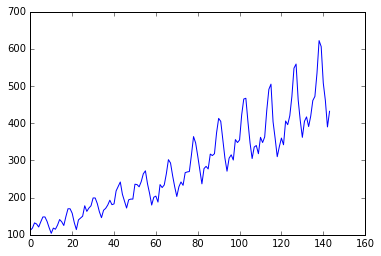• No products in the cart.

# 104.3.6 Creating Graphs in Python

##### Visualizing data in Python.

Link to the previous post : https://statinfer.com/104-3-5-box-plots-and-outlier-dectection-using-python/

In the last post we made box plot and understood how it is useful in detecting outliers.

In this post we will cover 3 most common plots for preliminary analysis.

• Scatter Plot
• Bar Chart
• Trend Chart

### Scatter Plot:

Scatter plots give us an indication on the relation between the two chosen variables.

Example:

```cars=pd.read_csv("datasets\\Cars Data\\Cars.csv",encoding = "ISO-8859-1")
cars.shape
```
`(428, 15)`
```cars.columns.values
```
```array(['Make', 'Model', 'Type', 'Origin', 'DriveTrain', 'MSRP', 'Invoice',
'EngineSize', 'Cylinders', 'Horsepower', 'MPG_City', 'MPG_Highway',
'Weight', 'Wheelbase', 'Length'], dtype=object)```
```cars['Horsepower'].describe()
```
```count    428.000000
mean     215.885514
std       71.836032
min       73.000000
25%      165.000000
50%      210.000000
75%      255.000000
max      500.000000
Name: Horsepower, dtype: float64```
```cars['MPG_City'].describe()
```
```count    428.000000
mean      20.060748
std        5.238218
min       10.000000
25%       17.000000
50%       19.000000
75%       21.250000
max       60.000000
Name: MPG_City, dtype: float64```
```import matplotlib.pyplot as plt
plt.scatter(cars.Horsepower,cars.MPG_City)
```
`<matplotlib.collections.PathCollection at 0xd272e10>`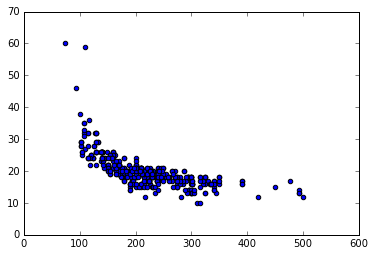### Practice : Creating Scatter Plots

• Dataset: “./Sporting_goods_sales/Sporting_goods_sales.csv”
• Draw a scatter plot between Average_Income and Sales. Is there any relation between two variables?
• Draw a scatter plot between Under35_Population_pect and Sales. Is there any relation between two?
```sports_data=pd.read_csv("datasets\\Sporting_goods_sales\\Sporting_goods_sales.csv",encoding = "ISO-8859-1")
```
Sr_no Avg_family_size Average_Income M_F_Gender_Ratio Un_emp_rate Under35_Population_pect Number_schools Sales
0 1 3 9305.306044 46.654268 2.587691 51.426218 395.379432 140870.7288
1 2 2 8907.622334 64.505029 2.731910 28.485052 316.503520 100305.7146
2 3 2 9846.602630 63.595331 4.269577 49.452727 359.077144 135474.6688
3 4 2 8871.731173 50.451251 3.124004 44.678507 346.833014 126349.5082
4 5 4 9891.047985 51.353801 2.004201 37.664024 329.034161 117434.7267
```# Draw a scatter plot between Average_Income and Sales. Is there any relation between two variables?

import matplotlib.pyplot as plt
plt.scatter(sports_data.Average_Income ,sports_data.Sales)
```
`<matplotlib.collections.PathCollection at 0xd2dbba8>`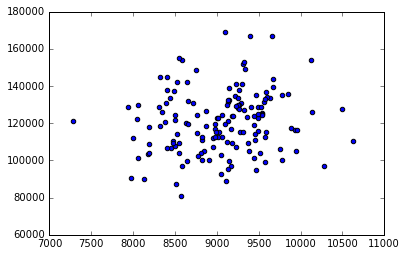```import matplotlib.pyplot as plt

plt.scatter(sports_data.Under35_Population_pect  ,sports_data.Sales)
```
`<matplotlib.collections.PathCollection at 0xd4f9eb8>`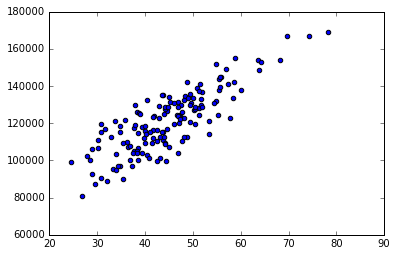### Bar Chart

Bar charts used to summarize the categorical variables

```freq=cars.Cylinders.value_counts()
freq.values
```
`array([190, 136,  87,   7,   3,   2,   1], dtype=int64)`
```freq.index
```
`Float64Index([6.0, 4.0, 8.0, 5.0, 12.0, 10.0, 3.0], dtype='float64')`
```import matplotlib.pyplot as plt

plt.bar(freq.index,freq.values)
```
`<Container object of 4 artists>`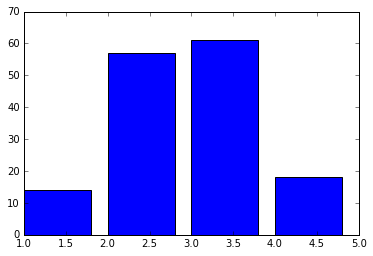### Practice : Bar Chart

• Dataset: “./Sporting_goods_sales/Sporting_goods_sales.csv”
• Create a bar chart summarizing the information on family size.
```freq=sports_data.Avg_family_size.value_counts()
freq.values
```
`array([61, 57, 18, 14], dtype=int64)`
```freq.index
```
`Int64Index([3, 2, 4, 1], dtype='int64')`
```import matplotlib.pyplot as plt
plt.bar(freq.index,freq.values)
```
`<Container object of 4 artists>`### Trend chart

• Trend chart is used for time series datasets
```AirPassengers=pd.read_csv("datasets\\Air Travel Data\\Air_travel.csv", encoding = "ISO-8859-1")
```
DATE AIR
0 JAN49 112
1 FEB49 118
2 MAR49 132
3 APR49 129
4 MAY49 121
```AirPassengers.columns.values
```
`array(['DATE', 'AIR'], dtype=object)`
```import matplotlib.pyplot as plt
plt.plot(AirPassengers.AIR)
```
`[<matplotlib.lines.Line2D at 0xd55ff98>]`